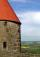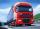# Volume - math word problems

#### Number of problems found: 696

• Cube 9What was the original edge length of the cube if after cutting 39 small cubes with an edge length 2 dm left 200 dm3?
• Prism 4 sidesThe prism has a square base with a side length of 3 cm. The diagonal of the sidewall of the prism/BG/is 5 cm. Calculate the surface of this prism in cm square and the volume in liters
• Triangular prismCalculate a triangular prism if it has a rectangular triangle base with a = 4cm and hypotenuse c = 50mm and height of the prism is 0.12 dm.
• An oilAn oil drum is cut in half. One half is used as a water trough. Use the dimensions; length 82cm, width 56cm to estimate the capacity of the water trough in liters.
• Pine woodFrom a trunk of pine 6m long and 35 cm in diameter with a carved beam with a cross-section in the shape of a square so that the square had the greatest content area. Calculate the length of the sides of a square. Calculate the volume in cubic meters of lu
• BottleA company wants to produce a bottle whose capacity is 1.25 liters. Find the dimensions of a cylinder that will be required to produce this 1.25litres if the hight of the cylinder must be 5 times the radius.
• PoolMr. Peter build a pool shape of a four-sided prism with rhombus base in the garden. Base edge length is 8 m, distance of the opposite walls of the pool is 7 m. Estimated depth is 144 cm. How many hectoliters of water consume Mr. Peter to fill the pool
• The poolThe pool has a volume of 40 m3 and the water temperature is 20 °C. How much water at 100 °C should we pour into the pool to increase the water temperature by 5 °C?
• PrismCalculate the surface area and volume of a prism with a body height h = 10 cm and its base has shape of a rhomboid with sides a = 5.8 cm, b = 3 cm and the distance of its two longer sides is w = 2.4 cm.The radius of the sphere we reduce by 1/3 of the original radius. How much percent does the volume and surface of the sphere change?
• Water levelHow high is the water in the swimming pool with dimensions of 37m in length and 15m in width, if an inlet valve is opened for 10 hours flowing 12 liters of water per second?
• Volume of coneFind the volume of a right circular cone-shaped building with a height of 9 cm and a radius base of 7 cm.
• Weight of airWhat is the weight of air in the living room measuring width 10 m length 3 m and height 3.3 m? Air density is ρ = 1.2 kg/m3.
• SnowSnow fell overnight layer of thickness 19 cm. In the morning I had to clear a path 69 m long and one meter wide. How many cubic meters of snow I clear? How many kilos was it? (1 m3 fresh snow weighs 350 kg)
• Reservoir 3How many cubic feet of water is stored in the reservoir that has a length of 200 feet, a width of 100 feet, an overflow depth of 32 feet, and a current water level of 24 feet?
• Plastic pipeCalculate weight of the plastic pipe with diameter d = 70 mm and length 380 cm if the wall thickness is 4 mm and the density of plastic is 1367 kg/m3.
• Truck of milkHow many hectoliters of ,,the box" milk fit in the truck, ake cargo size area is 2.8 m x 3 m x 17 m? A liter of milk in a box measuring 12 cm x 7 cm x 20 cm.
• Truncated pyramidHow many cubic meters is volume of a regular four-side truncated pyramid with edges one meter and 60 cm and high 250 mm?
• VintnerHow high can vintner fill keg with crushed red grapes if these grapes occupy a volume of 20 percent? Keg is cylindrical with a diameter of the base 1 m and a volume 9.42 hl. Start from the premise that says that fermentation will fill the keg (the number
• Tank and waterCylindrical tank were poured with 3.5 liters of water. If tank base diameter is 3 dm, how height is water level in?

Do you have an interesting mathematical word problem that you can't solve it? Submit a math problem, and we can try to solve it.

We will send a solution to your e-mail address. Solved examples are also published here. Please enter the e-mail correctly and check whether you don't have a full mailbox.

Please do not submit problems from current active competitions such as Mathematical Olympiad, correspondence seminars etc...